Publication date: 05/24/2021

## Agreement Statistic Option

Viewing the two response variables as two independent ratings of the n subjects, the Kappa coefficient equals +1 when there is complete agreement of the raters. When the observed agreement exceeds chance agreement, the Kappa coefficient is positive and its magnitude reflects the strength of agreement. Although unusual in practice, Kappa is negative when the observed agreement is less than chance agreement. The minimum value of Kappa is between -1 and 0, depending on the marginal proportions.

The Kappa coefficient is computed as follows: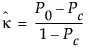where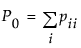and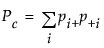Note that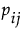is the proportion of subjects in the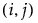th cell, such that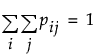.

The asymptotic variance of the simple kappa coefficient is estimated by the following: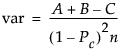where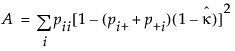,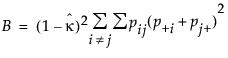and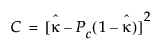See Cohen (1960) and Fleiss et al. (1969).

For Bowker’s test of symmetry, the null hypothesis is that the probabilities in the two-by-two table satisfy symmetry (pij=pji).